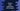# Python conver integer to hexadecimal using hex()## Python hex() method explanation with example:

hex() is one of the important method in Python. This method is used to convert any integer value to hexadecimal string value. If it is not an integer, or if it is an another object, it should have one index() method defined, that returns one integer value.

In this post, we will learn how to use hex method with examples.

## Definition of hex method:

hex method is defined as like below:

``hex(i)``

Here, i is an integer value and it returns one string, i.e. its hexadecimal value that is prefixed with 0x.

### Example of hex method:

Let’s take a look at how to use hex() with examples.

``````print(hex(100))
print(hex(-100))
print(hex(-30))``````

It will print the below output:

``````0x64
-0x64
-0x1e``````

### How to find the hex method for a floating point value:

We can also find the hex value for a floating point value. For that, we need to use float.hex() method. For example,

``print(hex(100.1))``

This will throw one TypeError:

``TypeError: 'float' object cannot be interpreted as an integer``

So, we can use float.hex to parse a floating point value:

``print(float.hex(100.1))``

It will print:

``0x1.9066666666666p+6``

### How to convert a hexadecimal value to integer:

We can also convert a hexadecimal value to integer. For that, we need to use the int() method. This method takes one hexadecimal value and convert that to an integer.

## Hex with a custom object:

As explained above, we need to define the index method that should return one integer value.

``````class SampleObject:
def __index__(self):
return 10

obj = SampleObject()

print(hex(obj))``````

Here, SampleObject is a class that has index() method that returns one integer value. If you run this code, it will print the below output:

``0xa``

Which is the hexadecimal value of 10.